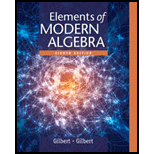# Whether the statement, “Every mapping on the nonempty set A is a relation” is true or false.### Elements Of Modern Algebra

8th Edition
Gilbert + 2 others
Publisher: Cengage Learning,
ISBN: 9781285463230### Elements Of Modern Algebra

8th Edition
Gilbert + 2 others
Publisher: Cengage Learning,
ISBN: 9781285463230

#### Solutions

Chapter 1.7, Problem 1TFE
To determine

## Whether the statement, “Every mapping on the nonempty set A is a relation” is true or false.

Expert Solution

Solution:

The statement, “Every mapping on the nonempty set A is a relation” is true.

### Explanation of Solution

Consider the statement, “Every mapping on the nonempty set A is a relation”.

Let A and B are non-empty sets. A subset f of A×B is a mapping from A to B if and only if for every aA there exists unique(one and only one) image bB such that (a,b)f

A relation on a nonempty set A is a nonempty set R of ordered pairs (x,y) of elements of x and y of A.

From the above definitions,

Every mapping is a relation.

Hence the statement, “Every mapping on the nonempty set A is a relation” is true.

### Want to see more full solutions like this?

Subscribe now to access step-by-step solutions to millions of textbook problems written by subject matter experts!

### Want to see more full solutions like this?

Subscribe now to access step-by-step solutions to millions of textbook problems written by subject matter experts!